Test: Modem Digital Modulation & Detection Techniques

# Test: Modem Digital Modulation & Detection Techniques - Electronics and Communication Engineering (ECE)

Test Description

## 10 Questions MCQ Test GATE ECE (Electronics) 2023 Mock Test Series - Test: Modem Digital Modulation & Detection Techniques

Test: Modem Digital Modulation & Detection Techniques for Electronics and Communication Engineering (ECE) 2023 is part of GATE ECE (Electronics) 2023 Mock Test Series preparation. The Test: Modem Digital Modulation & Detection Techniques questions and answers have been prepared according to the Electronics and Communication Engineering (ECE) exam syllabus.The Test: Modem Digital Modulation & Detection Techniques MCQs are made for Electronics and Communication Engineering (ECE) 2023 Exam. Find important definitions, questions, notes, meanings, examples, exercises, MCQs and online tests for Test: Modem Digital Modulation & Detection Techniques below.
Solutions of Test: Modem Digital Modulation & Detection Techniques questions in English are available as part of our GATE ECE (Electronics) 2023 Mock Test Series for Electronics and Communication Engineering (ECE) & Test: Modem Digital Modulation & Detection Techniques solutions in Hindi for GATE ECE (Electronics) 2023 Mock Test Series course. Download more important topics, notes, lectures and mock test series for Electronics and Communication Engineering (ECE) Exam by signing up for free. Attempt Test: Modem Digital Modulation & Detection Techniques | 10 questions in 30 minutes | Mock test for Electronics and Communication Engineering (ECE) preparation | Free important questions MCQ to study GATE ECE (Electronics) 2023 Mock Test Series for Electronics and Communication Engineering (ECE) Exam | Download free PDF with solutions
 1 Crore+ students have signed up on EduRev. Have you?
Test: Modem Digital Modulation & Detection Techniques - Question 1

### Assertion (A): Binary phase shift keying (BPSK) is the most efficient of the three digital modulation, i.e., ASK, FSK and PSK. Reason (R): In BPSK, phase of sinusoidal carrier is changed according to the data bit to be transmitted.

Detailed Solution for Test: Modem Digital Modulation & Detection Techniques - Question 1

BPSK is the most efficient of the three digital modulation, i.e. ASK, FSK and PSK because BPSK is used for high bit rates. Reason is also a true statement but not the correct explanation of assertion.

Test: Modem Digital Modulation & Detection Techniques - Question 2

### Which of the following modulation scheme is most affected by noise?

Test: Modem Digital Modulation & Detection Techniques - Question 3

### Match List-I with List-ll and select the correct answer using the codes given below the lists: List-I A. BPSK B. AM C. QPSK D. ASK  List-ll 1. A group of two binary bits is represented by one phase state. 2. A binary bit is represented by one phase state. 3. Carrier ON or OFF depending on whether ‘V or ‘0’ is to be transmitted. 4. Detected using envelope detector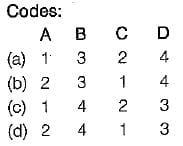Test: Modem Digital Modulation & Detection Techniques - Question 4

The bit rate of a digital communication system is 34 Mbps. The modulation scheme is QPSK. The baud rate of the system is

Detailed Solution for Test: Modem Digital Modulation & Detection Techniques - Question 4

In BPSK, baud rate is half the bit rate due to which there is more effective utilization of the available bandwidth of the transmission channel. Given, bit rate = 34 Mbps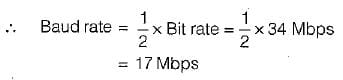Test: Modem Digital Modulation & Detection Techniques - Question 5

Consider the following statements;
1. BPSK has a bandwidth which is lower than that of a BFSK signal.
2. BPSK yields the maximum value of probability of error compared to ail the three digital modulation techniques i.e. ASK, FSK and PSK.
3. Binary FSK has the highest system complexity.
4. Binary ASK is demodulated using coherent detection while binary FSK and PSK are demodulated using envelope detection

Which of the statements given above are correct?

Detailed Solution for Test: Modem Digital Modulation & Detection Techniques - Question 5
• Statement-1 is correct.
• BPSK has the best performance of all the three digital modulation techniques in presence of noise, it yields the minimum value of probability of error compared to ASK, FSK and PSK. Hence, statement-2 is false.
• Statement-3 is correct since binary FSK has highest system complexity while binary ASK has least system complexity.
• Binary ASK is demodulated using envelope detection while binary FSK and PSK are demodulated using coherent detection.

Hence, statement-4 is fals

Test: Modem Digital Modulation & Detection Techniques - Question 6

Comparison of MSKand QPSK scheme shows that

Detailed Solution for Test: Modem Digital Modulation & Detection Techniques - Question 6

More energy (or power) is contained in the main lobe of MSK (about 99% of the total energy or power) as compared to the QPSK (about 90% of the total energy or power).

Test: Modem Digital Modulation & Detection Techniques - Question 7

In a digital continuous-time communication system, the bit rate of NRZ data stream is 1 Mbps and carrier frequency of transmission is 100 MHz. What is the bandwidth requirement of the channel in BPSK and QPSK systems respectively?

Detailed Solution for Test: Modem Digital Modulation & Detection Techniques - Question 7

The bit period of NRZ data stream is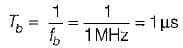In BPSK, each binary bit is a symbol, therefore symbol duration Ts = Tb = 1 μs.
∴ The bandwidth required for BPSK system is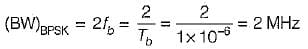In QPSK, we group two successive bits to form one symbol, therefore symbol duration,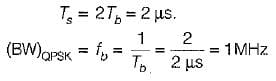Test: Modem Digital Modulation & Detection Techniques - Question 8

The error probability of QPSK is

Detailed Solution for Test: Modem Digital Modulation & Detection Techniques - Question 8

The error probability of QPSK is better (or lower) than that of BPSK due to which QPSK is used for very high bit rate data transmission.

Test: Modem Digital Modulation & Detection Techniques - Question 9

Bandwidth of BPSK is ___ than that of BFSK.

Detailed Solution for Test: Modem Digital Modulation & Detection Techniques - Question 9

Minimum bandwidth required in BPSK = 2fb.
Minimum bandwidth required in BFSK = 4fb.
Hence, bandwidth of BPSK is lower than that of BFSK.

Test: Modem Digital Modulation & Detection Techniques - Question 10

The error probability of QPSK is

Detailed Solution for Test: Modem Digital Modulation & Detection Techniques - Question 10

The error probability of QPSK is better (or lower) than that of BPSK due to which QPSK is used for very high bit rate data transmission.

## GATE ECE (Electronics) 2023 Mock Test Series

21 docs|263 tests
Information about Test: Modem Digital Modulation & Detection Techniques Page
In this test you can find the Exam questions for Test: Modem Digital Modulation & Detection Techniques solved & explained in the simplest way possible. Besides giving Questions and answers for Test: Modem Digital Modulation & Detection Techniques, EduRev gives you an ample number of Online tests for practice

## GATE ECE (Electronics) 2023 Mock Test Series

21 docs|263 tests(Scan QR code)